# Algebra for 4th Graders:CCSSM 4.NF.3d

##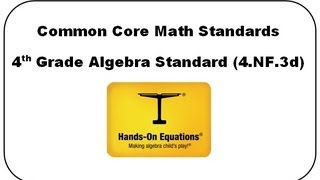By Henry Borenson

Use visual models and equations to represent and solve word problems involving addition and subtraction of fractions (4.NF.3d)# 4.NF.5 & 4.NF.6 Fractions and Decimals

##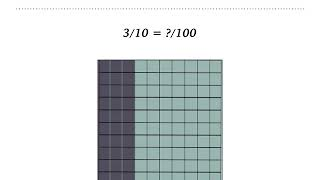By Tiffany Nickel

an understanding of fraction equivalence with denominators 10 and 100 as well as decimal notation.# Fractions with a Denominator of 10 and 100: 4.NF.5

##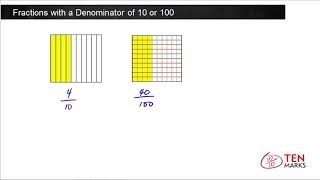By TenmarksAmazon

fractions with a denominator of 10 as a fraction with a denominator of 100# Fractions with a Denominator of 10 and 100: 4.NF.5

##By TenmarksAmazon

explains how to rewrite fractions with a denominator of 10 as a fraction with a denominator of 100.# Decimal Notation and Fractions: 4.NF.6

##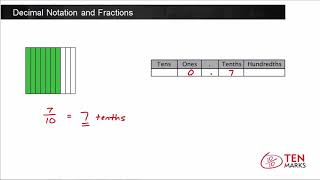By TenmarksAmazon

explains how to write fractions with denominators of 10 or 100 as a decimal# [4.NF.6-1.0] Simple Decimal Notation - Common Core Standard

##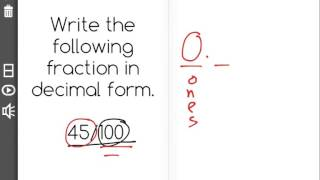By Freckle education

Use decimal notation for fractions with denominators 10 or 100# 4.NF.4.c - Fraction of a Set Word Problems (Singapore Math)

##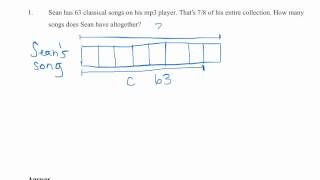By MathWithMrAlmeida

multiplying a fraction by a whole number to solve word problems# Multiplying fractions by whole numbers 4.NF.B.4 4.NF.4

##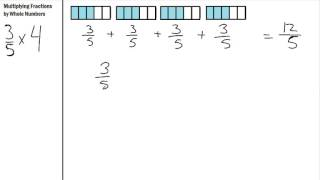By S Hart

Multiplying Fractions by Whole numbers using repeated addition# [4.NF.4a-1.0] Understanding Multiples - Common Core Standard

##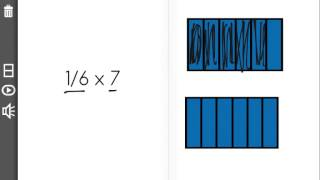By Freckle education

Understand a fraction a/b as a multiple of 1/b# [4.NF.4b-1.4] Multiplying Fractions - Common Core Standard

##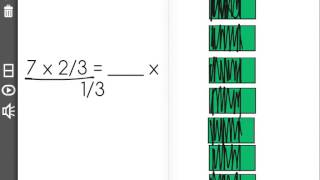By Freckle education

Understand a multiple of a/b as a multiple of 1/b# [4.NF.4b-1.0] Multiplying Fractions - Common Core Standard

##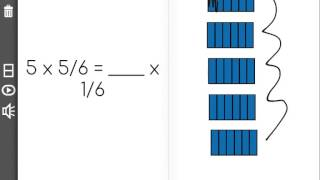By Freckle education

Understand a multiple of a/b as a multiple of 1/b, and use this understanding to multiply a fraction by a whole number# 4.NF.2-1.0] Compare Dissimilar Fractions - Common Core Standard

##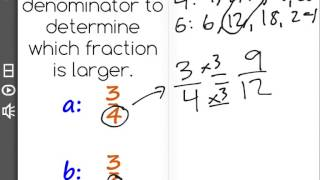By Freckle education

Compare two fractions with different numerators and different denominators# [4.NF.4c-1.0] Word Problems: Multiplying - Common Core Standard

##By Freckle education

Solve word problems involving multiplication of a fraction by a whole number, e.g., by using visual fractions models and equations to represent the problem.# [4.NF.3b-1.0] Decomposing Fractions - Common Core Standard

##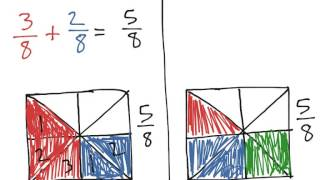By Freckle education

Decompose a fraction into a sum of fractions with the same denominator in more than one way# [4.NF.1-1.0] Generate Equivalent Fractions - Common Core Standard

##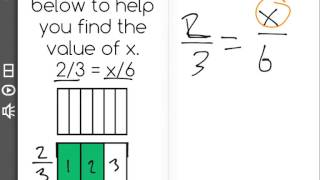By Freckle education

Explain why a fraction a/b is equivalent to a fraction (n x a) / (n x b) by using visual fraction models# [4.NF.7-1.0] Comparing Decimals - Common Core Standard - Practice Problem

##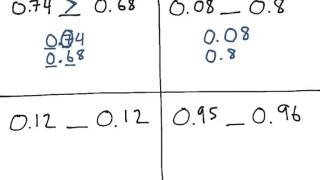By Freckle education

Compare two decimals to hundredths by reasoning about their size, recording the results with the symbols greater than, =, or less than, and justify the conclusions by using a visual fraction model# [4.NF.3a-1.0] Intro to Fraction Addition - Common Core Standard

##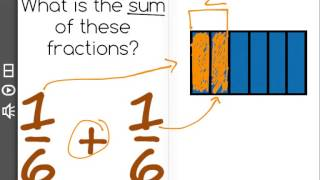By Freckle education

Understand addition and subtraction of fractions as joining and separating parts referring to the same whole# 4.NF.5

##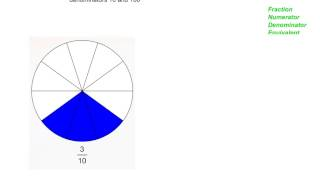By Benjamin Light

Express a fraction with denominator 10 as an equivalent fraction with denominator 100, and use this technique to add two fractions with respective denominators 10 and 100.# 4.nf.1

##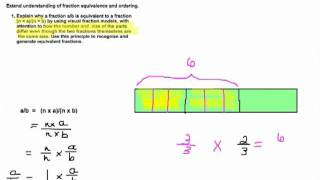By SEDL

The Common Core State Standards (CCSS) videos are designed to support states, schools, and teachers in the implementation of the CCSS. Each video is an audiovisual resource that focuses on one or more specific standards and usually includes examples/illustrations geared to enhancing understanding. The intent of each content-focused video is to clarify the meaning of the individual standard rather than to be a guide on how to teach each standard although the examples can be adapted for instructional use.# [4.NF.4a-1.0] Understanding Multiples - Common Core Standard - Practice Problem

##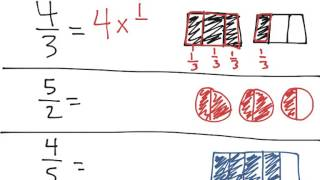By Freckle education

Understand a fraction a/b as a multiple of 1/b.